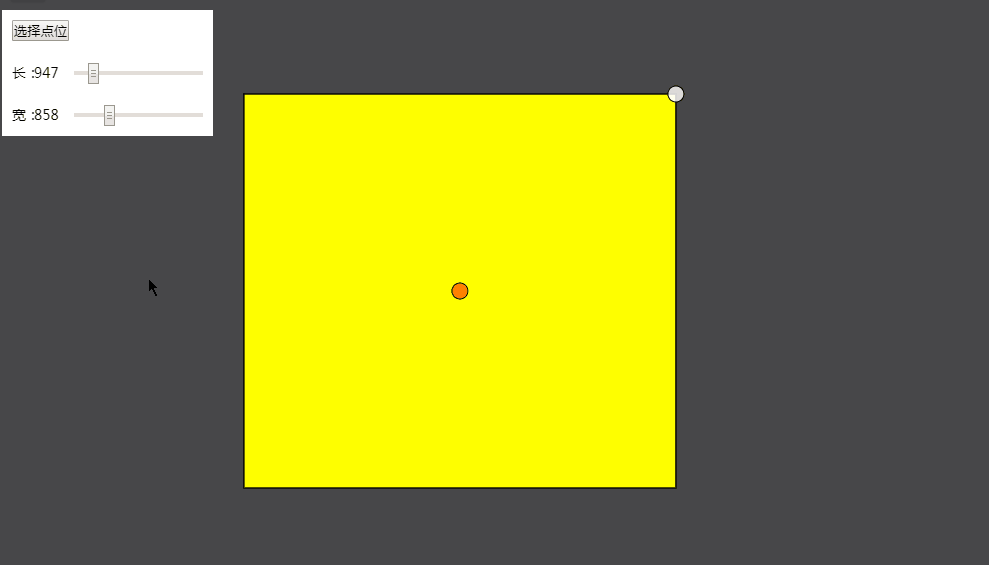# ArcGIS JavaScript 4. Generates a rectangular buffer at the point of X

2022-05-15 02:15:36Long, ya

Record your own ArcGIS JavaScript 4.x Realize to point (Point) The effect of generating rectangular buffer with arbitrary length and width for the center . The key is through the central point coordinates , Long , Width as a condition, calculate the coordinates of each vertex to draw a rectangular polygon (polygon) The graphic . The following figure shows the demonstration effect :Draw rectangular buffer related code :

``````/**
*  Method of drawing rectangular buffer
*  Center point -[lon,lat]
*  Long -width
*  wide -height
*/
function getRectangleBuffer (centerPt, width, height) {
var xieLineDis = Math.sqrt(width * width + height * height);
var Tdegs = deg(Math.atan(width / height)); // Radians to degrees
var coordTopLeft = distanceAngle({ lon: centerPt, lat: centerPt }, -Tdegs, xieLineDis / 2); // Top left coordinates
var coordTopRight = distanceAngle({ lon: centerPt, lat: centerPt }, Tdegs, xieLineDis / 2); // Upper right corner
var coordBottomLeft = distanceAngle({ lon: centerPt, lat: centerPt }, -(180 - Tdegs), xieLineDis / 2); // The lower left corner
var coordBootomRight = distanceAngle({ lon: centerPt, lat: centerPt }, 180 - Tdegs, xieLineDis / 2); // The lower right corner
// Construct a polygon Required circular array
var points = [
[coordTopLeft.lon, coordTopLeft.lat],
[coordTopRight.lon, coordTopRight.lat],
[coordBootomRight.lon, coordBootomRight.lat],
[coordBottomLeft.lon, coordBottomLeft.lat]
]
// The point in the upper right corner , Used to synchronously enlarge the rectangle
var scalePt = new Point({
longitude: coordTopRight.lon,
latitude: coordTopRight.lat,
spatialReference: view.spatialReference
})
var scaleSym = {
type: "simple-marker",
color: [266, 266, 266, 0.8]
}
scaleGp = new Graphic({
geometry: scalePt,
symbol: scaleSym,
attributes: {
name: "rectangleGp"
}
})
var polygon = new Polygon({
rings: points
})
var recsymbol = {
type: "simple-fill",
color: [267, 267, 0]
}
var rectangleGp = new Graphic({
geometry: polygon,
symbol: recsymbol
})
return rectangleGp;
}``````